# ML Aggarwal Class 10 Solutions for ICSE Maths Chapter 13 Similarity Ex 13.1

## ML Aggarwal Class 10 Solutions for ICSE Maths Chapter 13 Similarity Ex 13.1

ML Aggarwal Class 10 Solutions for ICSE Maths Chapter 13 Similarity Ex 13.1

Question 1.
State which pairs of triangles in the figure given below are similar. Write the similarity rule used and also write the pairs of similar triangles in symbolic form (all lengths of sides are in cm):Solution:Question 2.
It is given that ∆DEF ~ ∆RPQ. Is it true to say that ∠D = ∠R and ∠F = ∠P ? Why?
Solution:Question 3.
If in two right triangles, one of the acute angles of one triangle is equal to an acute angle of the other triangle, can you say that the two triangles are similar? Why?
Solution:Question 4.
In the given figure, BD and CE intersect each other at the point P. Is ∆PBC ~ ∆PDE? Give reasons for your answer.Solution:Question 5.
It is given that ∆ABC ~ ∆EDF such that AB = 5 cm, AC = 7 cm, DF = 15 cm and DE = 12 cm.
Find the lengths of the remaining sides of the triangles.Solution:Question 6.
(a) If ∆ABC ~ ∆DEF, AB = 4 cm, DE = 6 cm, EF = 9 cm and FD = 12 cm, then find the perimeter of ∆ABC.
(b) If ∆ABC ~ ∆PQR, Perimeter of ∆ABC = 32 cm, perimeter of ∆PQR = 48 cm and PR = 6 cm, then find the length of AC.
Solution: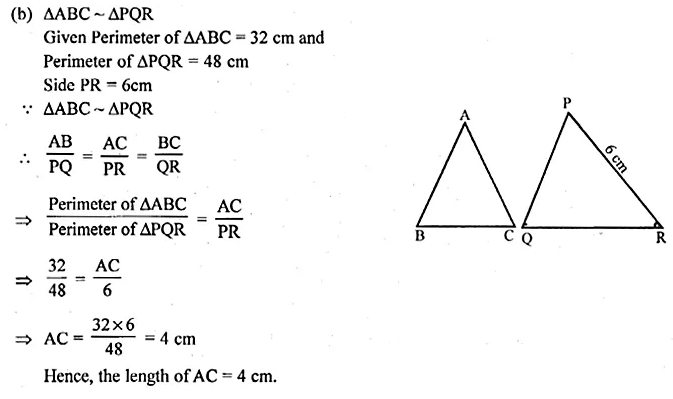Question 7.
Calculate the other sides of a triangle whose shortest side is 6 cm and which is similar to a triangle whose sides are 4 cm, 7 cm and 8 cm.
Solution:Question 8.
(a) In the figure given below, AB || DE, AC = , 3 cm, CE = 7.5 cm and BD = 14 cm. Calculate CB and DC.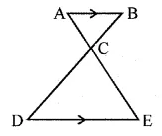(b) In the figure (2) given below, CA || BD, the lines AB and CD meet at G.
(i) Prove that ∆ACO ~ ∆BDO.
(ii) If BD = 2.4 cm, OD = 4 cm, OB = 3.2 cm and AC = 3.6 cm, calculate OA and OC.Solution: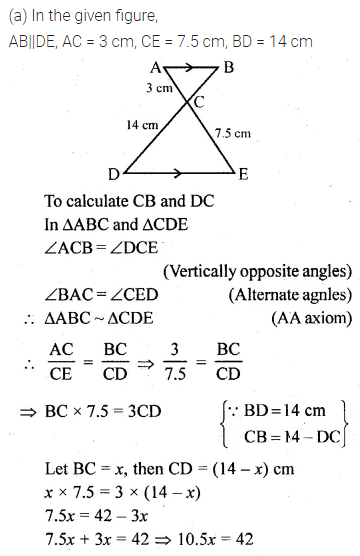Question 9.
(a) In the figure
(i) given below, ∠P = ∠RTS.
Prove that ∆RPQ ~ ∆RTS.
(b) In the figure (ii) given below,
∠ADC = ∠BAC. Prove that CA² = DC × BC.Solution:Question 10.
(a) In the figure (1) given below, AP = 2PB and CP = 2PD.
(i) Prove that ∆ACP is similar to ∆BDP and AC || BD.
(ii) If AC = 4.5 cm, calculate the length of BD.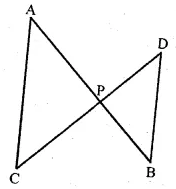(b) In the figure (2) given below,
(i) Prove that ∆s ABC and AED are similar.
(ii) If AE = 3 cm, BD = 1 cm and AB = 6 cm, calculate AC.(c) In figure (3) given below, ∠PQR = ∠PRS. Prove that triangles PQR and PRS are similar. If PR = 8 cm, PS = 4 cm, calculate PQ.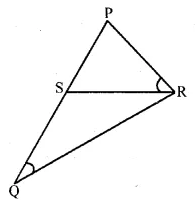Solution: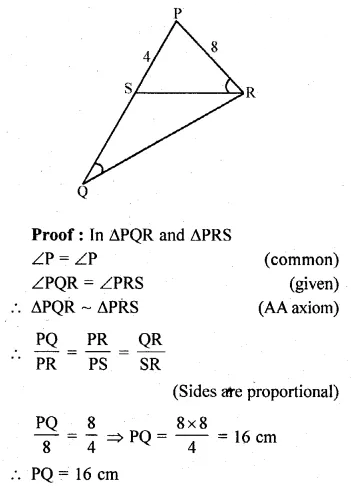Question 11.
In the given figure, ABC is a triangle in which AB = AC. P is a point on the side BC such that PM ⊥ AB and PN ⊥ AC. Prove that BM × NP = CN × MP.Solution:Question 12.
Prove that the ratio of the perimeters of two similar triangles is the same as the ratio of their corresponding sides.
Solution:Question 13.
In the given figure, ABCD is a trapezium in which AB || DC. The diagonals AC and BD intersect at O. Prove that $$\frac { AO }{ OC } =\frac { BO }{ OD }$$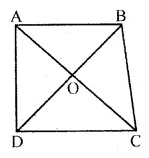Using the above result, find the value(s) of x if OA = 3x – 19, OB = x – 4, OC = x – 3 and OD = 4.
Solution: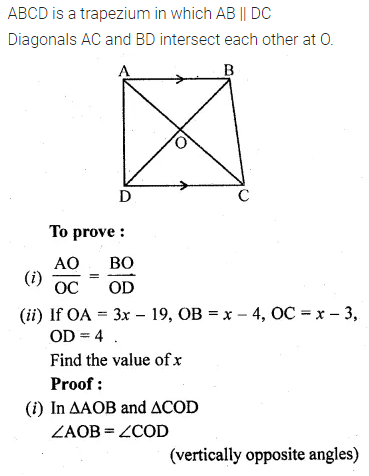Question 14.
In ∆ABC, ∠A is acute. BD and CE are perpendicular on AC and AB respectively. Prove that AB x AE = AC x AD.
Solution:Question 15.
In the given figure, DB ⊥ BC, DE ⊥ AB and AC ⊥ BC. Prove that $$\frac { BE }{ DE } =\frac { AC }{ BC }$$Solution:Question 16.
(a) In the figure (1) given below, E is a point on the side AD produced of a parallelogram ABCD and BE intersects CD at F. show that ∆ABE ~ ∆CFB.
(b) In the figure (2) given below, PQRS is a parallelogram; PQ = 16 cm, QR = 10 cm. L is a point on PR such that RL : LP = 2 : 3. QL produced meets RS at M and PS produced at N.
(i) Prove that triangle RLQ is similar to triangle PLN. Hence, find PN.
(ii) Name a triangle similar to triangle RLM. Evaluate RM.Solution:Question 17.
The altitude BN and CM of ∆ABC meet at H. Prove that
(i) CN . HM = BM . HN .
(ii) $$\frac { HC }{ HB } =\sqrt { \frac { CN.HN }{ BM.HM } }$$
(iii) ∆MHN ~ ∆BHC.
Solution: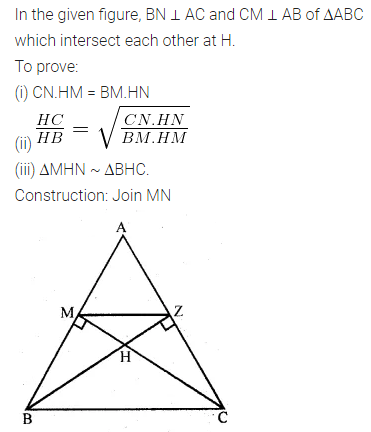Question 18.
In the given figure, CM and RN are respectively the medians of ∆ABC and ∆PQR. If ∆ABC ~ ∆PQR, prove that:
(i) ∆AMC ~ ∆PNR
(ii) $$\frac { CM }{ RN } =\frac { AB }{ PQ }$$
(iii) ∆CMB ~ ∆RNQ.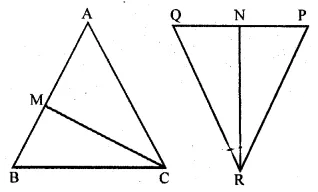Solution:Question 19.
In the given figure, medians AD and BE of ∆ABC meet at the point G, and DF is drawn parallel to BE. Prove that
(i) EF = FC
(ii) AG : GD = 2 : 1Solution:Question 20.
(a) In the figure given below, AB, EF and CD are parallel lines. Given that AB =15 cm, EG = 5 cm, GC = 10 cm and DC = 18 cm. Calculate
(i) EF
(ii) AC.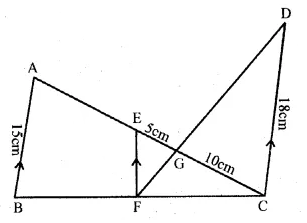(b) In the figure given below, AF, BE and CD are parallel lines. Given that AF = 7.5 cm, CD = 4.5 cm, ED = 3 cm, BE = x and AE = y. Find the values of x and y.Solution: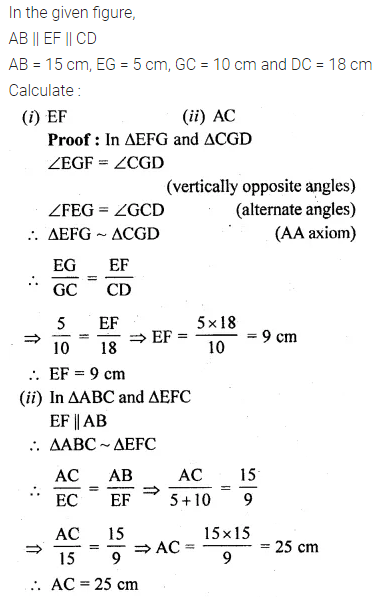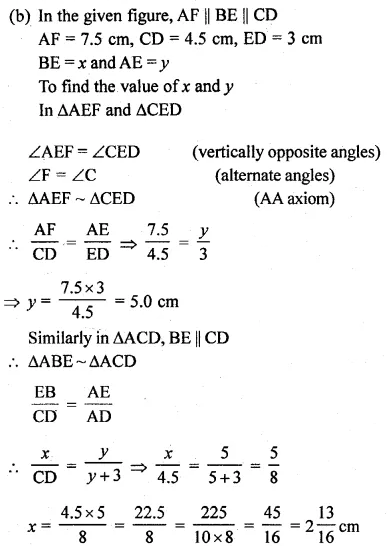Question 21.
In the given figure, ∠A = 90° and AD ⊥ BC If BD = 2 cm and CD = 8 cm, find AD.Solution: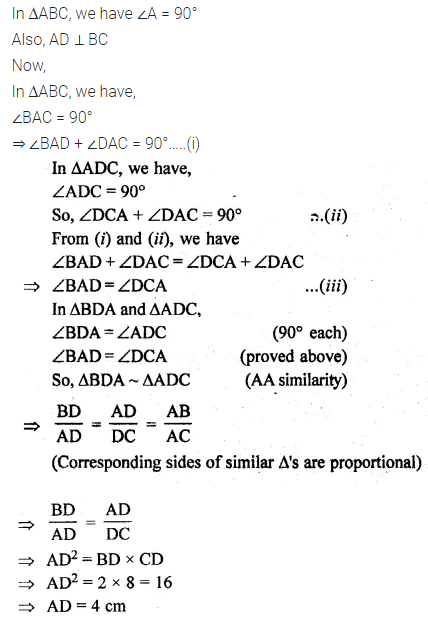Question 22.
A 15 metres high tower casts a shadow of 24 metres long at a certain time and at the same time, a telephone pole casts a shadow 16 metres long. Find the height of the telephone pole.
Solution: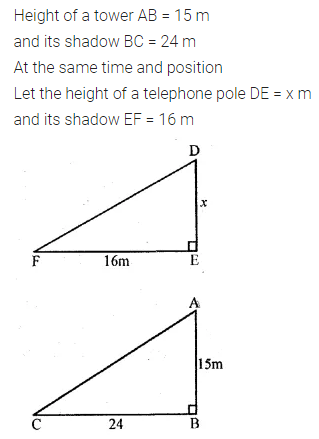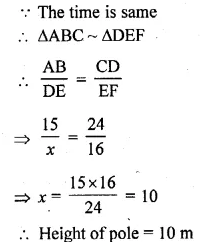Question 23.
A street light bulb is fixed on a pole 6 m above the level of the street. If a woman of height casts a shadow of 3 m, find how far she is away from the base of the pole?
Solution: